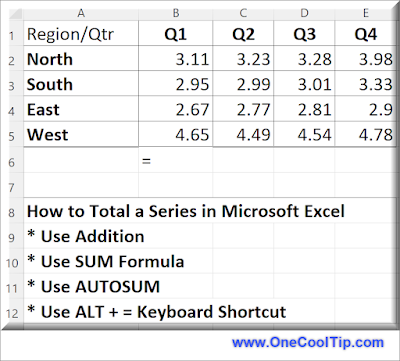## Monday, August 28, 2023

### Summing It Up: The Many Ways to Total in Microsoft Excel

Whether you’re balancing your checkbook or calculating the total cost of a project, summing a series of numbers is a common task.

But did you know that there are several ways to accomplish this?

Here's a Cool Tip:  Use the Excel keyboard shortcut.

To total, or sum, a series of numbers in Excel, you can use several methods:

• This is a very simple method to add the value of several cells containing numbers together.
• Use the Formula: =A1 + A2 + A3 + A4  where A1, A2, A3 and A4 are the cells containing the numbers you want to total.
• This works for a few cells but is unwieldly for a large set of numbers.

Use the SUM function:
• The SUM function is a built-in function in Excel that allows you to easily add up a range of cells.
• To use the SUM function, simply enter the formula =SUM(A1:A4) where A1:A4 is the range of cells containing the numbers you want to total.

Use AutoSum:
• AutoSum is a tool in Excel that automatically selects a column or row of numbers and calculates their sum.
• To use AutoSum, select the cell where you want to display the sum, then click on the AutoSum button in the toolbar on your screen.fig. 1 - Microsoft Excel Total Series

Super Cool Tip:

Use the Excel Keyboard Shortcut ALT + = to sum a series of numbers in a column or a row.

Enjoy!